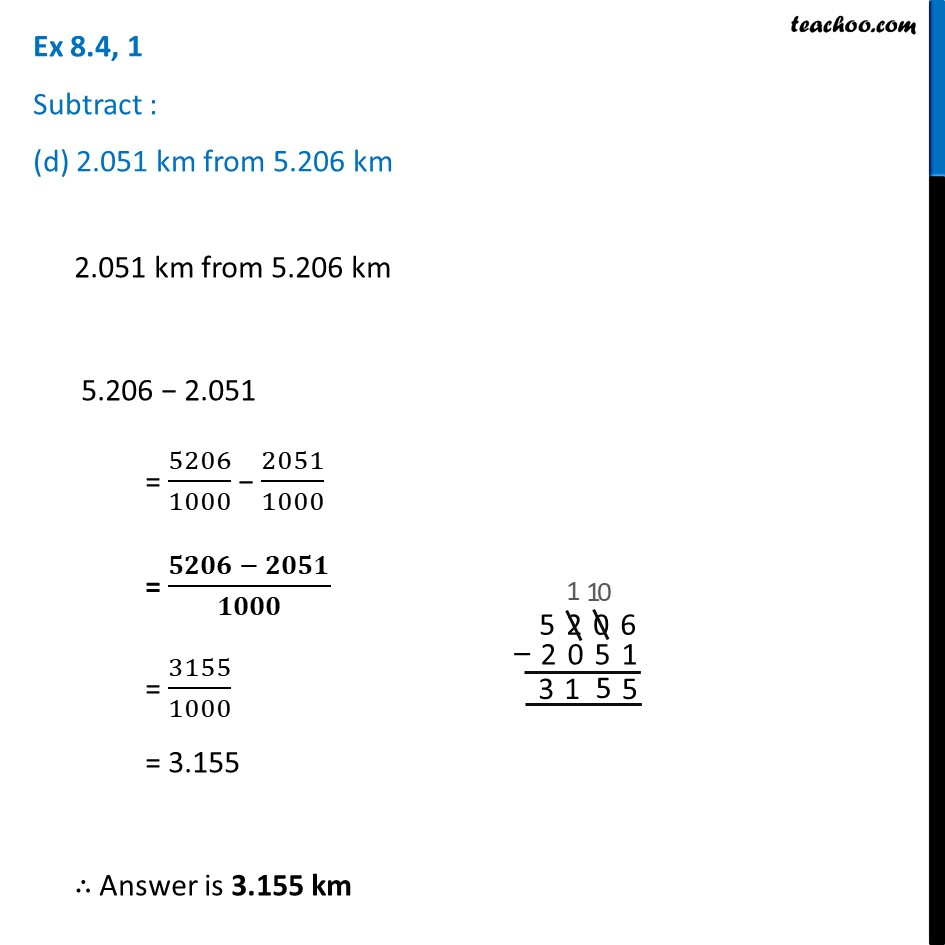Ex 8.4

Chapter 8 Class 6 Decimals
Serial order wiseLearn in your speed, with individual attention - Teachoo Maths 1-on-1 Class

### Transcript

Ex 8.4, 1 Subtract : (d) 2.051 km from 5.206 km2.051 km from 5.206 km 5.206 − 2.051 = 5206/1000 − 2051/1000 = (𝟓𝟐𝟎𝟔 − 𝟐𝟎𝟓𝟏)/𝟏𝟎𝟎𝟎 = 3155/1000 = 3.155 ∴ Answer is 3.155 km